The Band Structure of GaAs by the Empirical Pseudopotential Method

Kevin D. Welsher March 17, 2007

(Submitted as coursework for AP272, Stanford University, Winter 2007)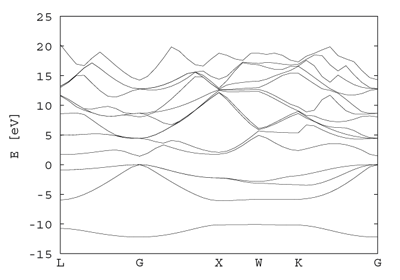Fig. 1: Band structure of GaAs calculated using the pseudo- potential as described in the text.

In the calculation of a band structure, the complexity (and therefore calculation time) increase dramatically with increasing atomic number. For instance, when calculating the band structure of carbon in the form of diamond, one only has to consider the 1s, 2s and 2p atomic orbitals as contributors to the band structure of the solid. For Silicon, we need to also include the 3s and 3p states into the calculation. This increases calculation time by both increasing the number and complexity of states involved, since the new "valence" states must be orthogonal to the "core" states, resulting in more complicated wavefunctions which oscillate near the nucleus.

One way to to fix this problem is to simplify it in such a way that we only need to consider the valence states. To do this we can replace the actual potential by a pseudopotential. This pseudopotential should incorporate the following effects:

 1 The effective nuclear potential seen by the valence electrons due to screening by the core electrons. 2 The electron-electron repulsion from the core states on the valence states.

Assuming we know the form of this pseudopotential we can set up a new wave equation: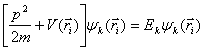Since the pseudopotential should be a small perturbation on the free electron band structure, we can use a linear combination of plane waves as a solution to the pseudo-wave equation. It should also be noted that a plane wave of momentum k has the same symmetry as a plane wave of momentum k+g, where g is a reciprocal lattice vector. The wavefunction can be expressed as follows: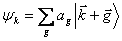Form Factor Value in Rydergs
V3s -0.23
V8s 0.01
V11s 0.06
V3a 0.07
V4a 0.05
V11a 0.01
Table 1: Pseudopotential form factors for GaAs from the literature.1

Each ket represents a plane wave with the indicated wave vector. It is easy to see that the size of the Hamiltonian we need to diagonalize depends directly on the number of reciprocal lattice vectors, g, we choose to include. When we construct the matrix of the Hamiltonian the form of the matrix elements of the pseudopotential are given by: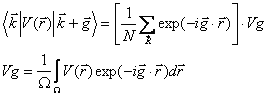From this definition, only matrix elements where g is a reciprocal lattice vector are nonzero. In the case of GaAs, the unit cell is comprised of two different types of atoms, denoted by a and b. It is a straightforward simplification to show that2: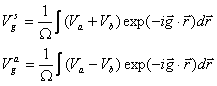After further simplification, we find: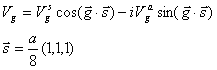Vgs and Vga stand for the symmetric and antisymmetric form factors of the pseudopotential. The index, g, is defined by the magnitude of the reciprocal lattice vector difference between two plane waves for a given matrix element. For a tetrahedrally bonded solid such as GaAs, the reciprocal lattice vectors only have magnitudes of and so on 0, 3, 4, 8, and 11. For our purposes, we will only consider form factors up to 11. The potential at |g|=0 is a constant, so it is taken to be zero or adjusted to some other appropriate value. For solids with a zinc-blende structure, V4s=V8a=0.

Since this is an empirical method, the band structure should be solved at high symmetry points to determine measureable quantities, such as the band gap. This value is then compared to experimentally determined values. If they do not match to within the desired degree, the form factors are adjusted and the process is repeated. Lucky for us, people have already done this.1 The values of the form factors we will use can be see in Table 1.

The value of that lattice constant, a, was also taken from Ref. 1, with a value of 5.64 angstroms. As stated before, the size of the Hamiltonian is determined purely by the number of plane waves used to generate it. Take for instance the &Gamma [k=(0,0,0)] point. The plane waves will of the form: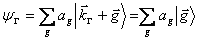We construct a complete set of reciprocal lattice vectors by the following definition: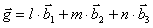l, m, and n are integers of the set {-2,-1,0,1,2} and b1, b2, and b3 are the reciprocal lattice vectors. By cycling the values of l, m, and n, we can get a 125x125 Hamiltonian matrix to be diagonalized. A unique method for generating the necessary reciprocal lattice vectors can be found in Ref. 3. The Hamiltonian is now given by: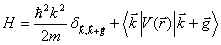The source code for the construction of the Hamiltonian can be found here. By diagonalizing the matrix, we get the energy levels at a given symmetry point of the solid. Starting with the L point, this matrix was solved at linearly spaced intervals between high symmetry points as shown below.

L=2&pi/a(1/2,1/2,1/2)→&Gamma=2&pi/a(0,0,0)→X=2&pi/a(1,0,0)→W=2&pi/a(1,1/2,0)→K=2&pi/a(3/4,3/4,0)→ &Gamma=2&pi/a(0,0,0)

A total of 40 points were calculated, taking less than one minute. The matrix diagonalization code was obtained from www.netlib.org/eispack. The actual code used in this calculation can be found here. The results were plotted in gnuplot, the input code for which can be found here. For ease of viewing, only the lowest 15 (of 125) eigenvalues are plotted at each symmetry point.

© 2007 Kevin D. Welsher. The author grants permission to copy, distribute and display this work in unaltered form, with attribution to the author, for noncommercial purposes only. All other rights, including commercial rights, are reserved to the author.

References

 M. L. Cohen and T.K. Bergstresser, "Band Structures and Pseudopotential Form Factors for Fourteen Semiconductors of the Diamond and Zinc-blende Structures," Phys. Rev. 141, 2 (1966).

 P. Y. Yu and M. Cardona, Fundamentals of Semiconductors: Physics and Materials Properties; (Springer, 2005).

 A. J. Danner, An introduction to the empirical pseudopotential method, http://vcsel.micro.uiuc.edu/~adanner/pseudopotential.htm (accessed 3/5/07).